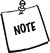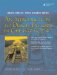# Selection Statements

Every programming language has at least one control structure that allows the flow of the program to branch depending on the outcome of a boolean condition. In C and C++ we have if and switch . The if statement typically has the following form.

```if(boolExpression)
statement
```

It can have an optional else attached.

```if(boolExpression)
statement1
else
statement2
```

Conditional statements can be nested, which means that they can get quite complicated. A very important rule to keep in mind is that an else or else if clause is activated when the boolExpression of the immediately preceding open if evaluates to false. This can be confusing when your program logic allows you to omit some else clauses. Consider the following badly indented example, where x is an int.

```if (x>0)
if (x > 100)
cout << "x is over a hundred";
else
if (x == 0) // no! this cannot be true -the indentation is misleading
cout << "x is 0";
else
cout << "x is negative"; // no! x is between 1 and 100 inclusive!
```

We can clarify and repair this logic with braces.

```if (x>0) {
if (x > 100)
cout << "x is over a hundred";
}
else
if (x == 0) // now this is possible.
cout << "x is 0";
else
cout << "x is negative";
```

An if without an else can be closed by enclosing the if statement in braces {}, making it a compound statement.

switch is another branching construct, which permits the execution of different code depending the value of a parameter.

```switch(integralExpression) {
case value1:
statement1;
break;
case value2:
statement2;
break;
...
case valuen:
statementn;
break;
default:
defaultStatement;
}
nextStatement;
```

The switch statement is a computed goto statement. Each case is followed by a unique case label value, which is compared to the integralExpression.

When the switch causes a jump to a case label whose value matches the integralExpression, statements are executed from that point on until the end of the switch block or a branching statement (e.g., break) is reached.

The optional default label is the jump destination when the integralExpression does not match any case label value. If default is omitted, and no matching case label exists, then the switch block is skipped.

The integralExpression must be an expression that evaluates to an integer. Each case label, except default, must be an integer constant.

 case labels are not the same as goto labels that are used as destinations for the infamous goto statement. goto labels must be identifiers. In particular, they cannot be integers.

Any switch statement such as the one above can be rewritten as a long if ... else statement. However, the runtime performance of a switch is considerably better because it requires only a single comparison and performs only one branch.

```if(integralExpression == value1)
statement1;
else if(integralExpression == value2)
statement2;
...
else if(integralExpression == valuen)
statementn;
else
defaultStatement;
```Long compound conditional statements and switch statements should to be avoided in object-oriented programming (unless they are isolated in factory code), because they tend to make functions complex and hard to maintain. If each case can be rewritten as a method of a different class, you can use the Strategy pattern (and the virtual table) instead of writing your own switch statement.

Exercise: Selection Statements

Be the computer and predict the output of the program in Exercise 21.1. Then run it and compare your predicted output with the output produced by the computer.

Example 21.1. src/early-examples/nestedif.cpp

 ```#include using namespace std; void nestedif1 () { int m = 5, n = 8, p = 11; if (m > n) if (p > n) cout << "red" << endl; else cout << "blue" << endl; } void nestedif2() { int m = 5, n = 8, p = 11; if (m > n) { if (p > n) cout << "red" << endl; } else cout << "blue" << endl; } int main() { nestedif1(); nestedif2(); return 0; } ```An Introduction to Design Patterns in C++ with Qt 4
ISBN: 0131879057
EAN: 2147483647
Year: 2004
Pages: 268